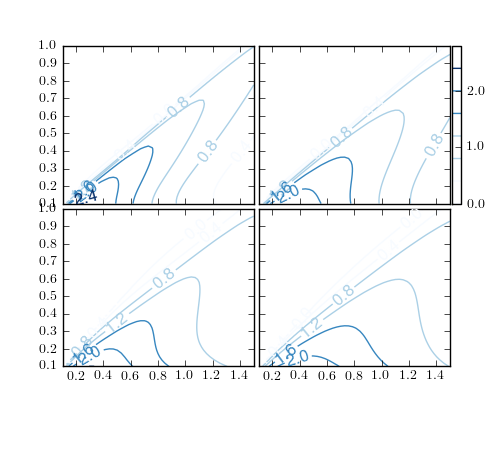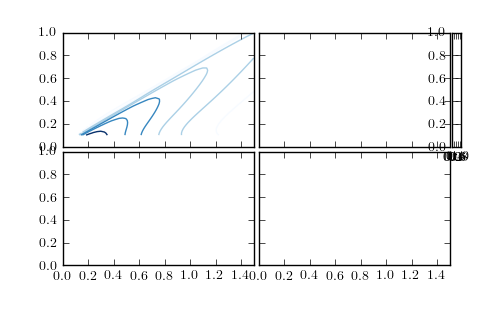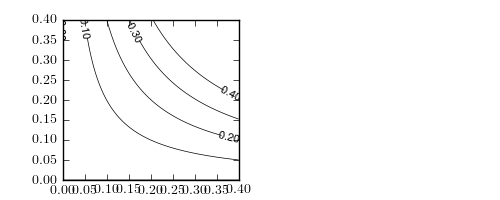# contour's clabels overlap each other in an ImageGrid

Hey guys,

I was hoping someone could provide a hint for how I should go about correcting this. As you can see, locations for the inline labels for
contours in this image are unfortunately chosen, and they overlap with one another. If I set manual to 'True' then I get this weird behavior
where I can only choose labels in the top left grid and nothing is shownin any of the other grids.

I am using a 2x2 Image Grid (I get the same problem when using gridspec instead of image grid), and loadingcontour data (2x2 array) from a file.

RonCan you post an simple self-contained script that reproduces your problem?
I just tried something similar but could not reproduces your problem.
Here is what I did,
Also, what version of matplotlb are you using?

Regards,

-JJ

import matplotlib.pyplot as plt
import numpy as np

from mpl_toolkits.axes_grid1 import ImageGrid

fig = plt.figure(1)

arr = np.arange(100).reshape((10, 10))

grid = ImageGrid(fig, 111, (2, 2))

cntr0 = grid.contour(arr)
cntr1 = grid.contour(arr)
cntr2 = grid.contour(arr)
cntr3 = grid.contour(arr)

plt.clabel(cntr0, manual=True)
plt.clabel(cntr1, manual=True)
plt.clabel(cntr2, manual=True)
plt.clabel(cntr3, manual=True)

···

On Thu, Aug 18, 2011 at 1:10 PM, R. O'Gara <ronogara@...9...> wrote:

Hey guys,

I was hoping someone could provide a hint for how I should go about correcting this. As you can see, locations for the inline labels for
contours in this image are unfortunately chosen, and they overlap with one another. If I set manual to 'True' then I get this weird behavior
where I can only choose labels in the top left grid and nothing is shownin any of the other grids.

I am using a 2x2 Image Grid (I get the same problem when using gridspec instead of image grid), and loadingcontour data (2x2 array) from a file.

Ron
------------------------------------------------------------------------------
user administration capabilities and model configuration. Take
the hassle out of deploying and managing Subversion and the
tools developers use with it. http://p.sf.net/sfu/wandisco-d2d-2
_______________________________________________
Matplotlib-users mailing list
Matplotlib-users@lists.sourceforge.net
https://lists.sourceforge.net/lists/listinfo/matplotlib-users

There is a combination of effects that make my contour labels placed in unfortunate positions. Here is a self-contained example (somewhat different from issue I originally explained, but illustrates the fact clabels are not where I'd like them to be)

import numpy as np
import matplotlib as mpl
ff = 10
params = {
'text.usetex': True,
'axes.labelsize': ff,
'axes.fontsize': ff,
'legend.fontsize' : ff,
'xtick.labelsize' : ff,
'ytick.labelsize' : ff,
'figure.dpi': 150,
'figure.figsize' : [5,2],
}
mpl.rcParams.update(params)
import matplotlib.pyplot as plt
import matplotlib.gridspec as gridspec

gs = gridspec.GridSpec(1,2)

ax = plt.subplot(gs)

xi = np.linspace(0,0.4,100)
yi = np.linspace(0,0.4,100)
xx,yy = np.meshgrid(xi,yi)
zi = np.sin(5*xx*yy)

v = np.arange(0.0, 0.5, 0.1)
cplot = ax.contour(xi, yi, zi, v, linewidths=0.5, colors='k')
ax.clabel(cplot, v,
fontsize = 8,
inline=True,
fmt = "%0.2f",
)
ax.axis([np.min(xi),np.max(xi),np.min(yi),np.max(yi)])
plt.show()

The end result is that contour labels are placed almost outside of the grid, with most of the area in the center being blank. I am pretty sure it has to do with the way rcParams are set, but I have no idea why. Params I do need to set are text.usetex, figure.dpi, and figure.figsize. I am using matplotlib 1.0.1.···

----- Original Message -----
From: Jae-Joon Lee <lee.j.joon@...287...>
To: R. O'Gara <ronogara@...9...>
Cc: "matplotlib-users@lists.sourceforge.net" <matplotlib-users@...1753...forge.net>
Sent: Monday, August 22, 2011 11:56 PM
Subject: Re: [Matplotlib-users] contour's clabels overlap each other in an ImageGrid

Can you post an simple self-contained script that reproduces your problem?
I just tried something similar but could not reproduces your problem.
Here is what I did,
Also, what version of matplotlb are you using?

Regards,

-JJ

import matplotlib.pyplot as plt
import numpy as np

from mpl_toolkits.axes_grid1 import ImageGrid

fig = plt.figure(1)

arr = np.arange(100).reshape((10, 10))

grid = ImageGrid(fig, 111, (2, 2))

cntr0 = grid.contour(arr)
cntr1 = grid.contour(arr)
cntr2 = grid.contour(arr)
cntr3 = grid.contour(arr)

plt.clabel(cntr0, manual=True)
plt.clabel(cntr1, manual=True)
plt.clabel(cntr2, manual=True)
plt.clabel(cntr3, manual=True)

On Thu, Aug 18, 2011 at 1:10 PM, R. O'Gara <ronogara@...9...> wrote:

Hey guys,

I was hoping someone could provide a hint for how I should go about correcting this. As you can see, locations for the inline labels for
contours in this image are unfortunately chosen, and they overlap with one another. If I set manual to 'True' then I get this weird behavior
where I can only choose labels in the top left grid and nothing is shownin any of the other grids.

I am using a 2x2 Image Grid (I get the same problem when using gridspec instead of image grid), and loadingcontour data (2x2 array) from a file.

Ron
------------------------------------------------------------------------------
user administration capabilities and model configuration. Take
the hassle out of deploying and managing Subversion and the
tools developers use with it. http://p.sf.net/sfu/wandisco-d2d-2
_______________________________________________
Matplotlib-users mailing list
Matplotlib-users@lists.sourceforge.net
https://lists.sourceforge.net/lists/listinfo/matplotlib-users

Hi,

I think this is partly because the current clabel implementation of
matpltolib does not correctly account the dpi setting. I opened a pull
request I think fix this problem. Please try that fix if you can.

https://github.com/matplotlib/matplotlib/pull/453

Otherwise, I think you can workaround this by using the default dpi
(72 or similar).

Regards,

-JJ

···

On Fri, Sep 2, 2011 at 11:28 PM, R. O'Gara <ronogara@...9...> wrote:

There is a combination of effects that make my contour labels placed in unfortunate positions. Here is a self-contained example (somewhat different from issue I originally explained, but illustrates the fact clabels are not where I'd like them to be)

import numpy as np
import matplotlib as mpl
ff = 10
params = {
'text.usetex': True,
'axes.labelsize': ff,
'axes.fontsize': ff,
'legend.fontsize' : ff,
'xtick.labelsize' : ff,
'ytick.labelsize' : ff,
'figure.dpi': 150,
'figure.figsize' : [5,2],
}
mpl.rcParams.update(params)
import matplotlib.pyplot as plt
import matplotlib.gridspec as gridspec

gs = gridspec.GridSpec(1,2)

ax = plt.subplot(gs)

xi = np.linspace(0,0.4,100)
yi = np.linspace(0,0.4,100)
xx,yy = np.meshgrid(xi,yi)
zi = np.sin(5*xx*yy)

v = np.arange(0.0, 0.5, 0.1)
cplot = ax.contour(xi, yi, zi, v, linewidths=0.5, colors='k')
ax.clabel(cplot, v,
fontsize = 8,
inline=True,
fmt = "%0.2f",
)
ax.axis([np.min(xi),np.max(xi),np.min(yi),np.max(yi)])
plt.show()

The end result is that contour labels are placed almost outside of the grid, with most of the area in the center being blank. I am pretty sure it has to do with the way rcParams are set, but I have no idea why. Params I do need to set are text.usetex, figure.dpi, and figure.figsize. I am using matplotlib 1.0.1.

----- Original Message -----
From: Jae-Joon Lee <lee.j.joon@...287...>
To: R. O'Gara <ronogara@...9...>
Cc: "matplotlib-users@lists.sourceforge.net" <matplotlib-users@...1544...ceforge.net>
Sent: Monday, August 22, 2011 11:56 PM
Subject: Re: [Matplotlib-users] contour's clabels overlap each other in an ImageGrid

Can you post an simple self-contained script that reproduces your problem?
I just tried something similar but could not reproduces your problem.
Here is what I did,
Also, what version of matplotlb are you using?

Regards,

-JJ

import matplotlib.pyplot as plt
import numpy as np

from mpl_toolkits.axes_grid1 import ImageGrid

fig = plt.figure(1)

arr = np.arange(100).reshape((10, 10))

grid = ImageGrid(fig, 111, (2, 2))

cntr0 = grid.contour(arr)
cntr1 = grid.contour(arr)
cntr2 = grid.contour(arr)
cntr3 = grid.contour(arr)

plt.clabel(cntr0, manual=True)
plt.clabel(cntr1, manual=True)
plt.clabel(cntr2, manual=True)
plt.clabel(cntr3, manual=True)

On Thu, Aug 18, 2011 at 1:10 PM, R. O'Gara <ronogara@...9...> wrote:

Hey guys,

I was hoping someone could provide a hint for how I should go about correcting this. As you can see, locations for the inline labels for
contours in this image are unfortunately chosen, and they overlap with one another. If I set manual to 'True' then I get this weird behavior
where I can only choose labels in the top left grid and nothing is shownin any of the other grids.

I am using a 2x2 Image Grid (I get the same problem when using gridspec instead of image grid), and loadingcontour data (2x2 array) from a file.